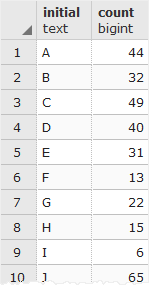# PostgreSQL Tutorial: LEFT Function: Get First N Characters in a String

September 19, 2023

The PostgreSQL `LEFT()` function returns the first `n` characters in the string.

## Syntax

The following illustrates the syntax of the PostgreSQL `LEFT()` function:

``````LEFT(string, n)
``````

## Arguments

The PostgreSQL `LEFT()` function requires two arguments:

1) `string`

is a string from which a number of the leftmost characters returned.

2) `n`

is an integer that specifies the number of left-most characters in the string should be returned.

If `n` is negative, the `LEFT()` function returns the leftmost characters in the string but last `|n|` (absolute) characters.

## Return value

The PostgreSQL `LEFT()` function returns the first `n` characters in a string.

## Examples

Let’s look at some examples of using the `LEFT()` function.

The following example shows how to get the first character of a string `'ABC'`:

``````SELECT LEFT('ABC', 1);
``````

The result is

`````` left
------
A
(1 row)
``````

To get the first two characters of the string ‘ABC’, you use 2 instead of 1 for the `n` argument:

``````SELECT LEFT('ABC', 2);
``````

Here is the result:

`````` left
------
AB
(1 row)
``````

The following statement demonstrates how to use a negative integer:

``````SELECT LEFT('ABC', -2);
``````

In this example, n is -2, therefore, the `LEFT()` function return all character except the last 2 characters, which results in:

`````` left
------
A
(1 row)
``````

See the following customer table in the sample database:

The following statement uses the `LEFT()` function to get the initials and the `COUNT()` function to return the number of customers for each initial.

``````SELECT LEFT(first_name, 1) initial,
COUNT(*)
FROM customer
GROUP BY initial
ORDER BY initial;
``````

In this example, first, the `LEFT()` function returns initials of all customers. Then, the `GROUP BY` clause groups customers by their initials. Finally, the `COUNT()` function returns the number of customer for each group.## Remarks

If you want to get the `n` rightmost characters, please see the `RIGHT()` function for the details.

In this tutorial, you have learned how to use the PostgreSQL `LEFT()` function to get the n left-most characters in a string.